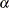### FORECAST Statement

FORECAST options ;

The FORECAST statement generates forecast values for a time series by using the parameter estimates produced by the previous ESTIMATE statement. See the section Forecasting Details for more information about calculating forecasts.

The following options can be used in the FORECAST statement:

ALIGN=option

controls the alignment of SAS dates used to identify output observations. The ALIGN= option allows the following values: BEGINNING|BEG|B, MIDDLE|MID|M, and ENDING|END|E. BEGINNING is the default.

ALPHA=n

sets the size of the forecast confidence limits. The ALPHA= value must be between 0 and 1. When you specify ALPHA=, the upper and lower confidence limits have aconfidence level. The default is ALPHA=0.05, which produces 95% confidence intervals. ALPHA values are rounded to the nearest hundredth.

BACK=n

specifies the number of observations before the end of the data where the multistep forecasts are to begin. The BACK= option value must be less than or equal to the number of observations minus the number of parameters.

The default is BACK=0, which means that the forecast starts at the end of the available data. The end of the data is the last observation for which a noise value can be calculated. If there are no input series, the end of the data is the last nonmissing value of the response time series. If there are input series, this observation can precede the last nonmissing value of the response variable, since there may be missing values for some of the input series.

ID=variable

names a variable in the input data set that identifies the time periods associated with the observations. The ID= variable is used in conjunction with the INTERVAL= option to extrapolate ID values from the end of the input data to identify forecast periods in the OUT= data set.

If the INTERVAL= option specifies an interval type, the ID variable must be a SAS date or datetime variable with the spacing between observations indicated by the INTERVAL= value. If the INTERVAL= option is not used, the last input value of the ID= variable is incremented by one for each forecast period to extrapolate the ID values for forecast observations.

INTERVAL=interval
INTERVAL=n

specifies the time interval between observations. See Chapter 4: Date Intervals, Formats, and Functions, for information about valid INTERVAL= values.

The value of the INTERVAL= option is used by PROC ARIMA to extrapolate the ID values for forecast observations and to check that the input data are in order with no missing periods. See the section Specifying Series Periodicity for more details.

specifies the number of multistep forecast values to compute. For example, if LEAD=10, PROC ARIMA forecasts for ten periods beginning with the end of the input series (or earlier if BACK= is specified). It is possible to obtain fewer than the requested number of forecasts if a transfer function model is specified and insufficient data are available to compute the forecast. The default is LEAD=24.

NOOUTALL

includes only the final forecast observations in the OUT= output data set, not the one-step forecasts for the data before the forecast period.

NOPRINT

suppresses the normal printout of the forecast and associated values.

OUT=SAS-data-set

writes the forecast (and other values) to an output data set. If OUT= is not specified, the OUT= data set specified in the PROC ARIMA statement is used. If OUT= is also not specified in the PROC ARIMA statement, no output data set is created. See the section OUT= Data Set for more information.

PRINTALL

prints the FORECAST computation throughout the whole data set. The forecast values for the data before the forecast period (specified by the BACK= option) are one-step forecasts.

SIGSQ=value

specifies the variance term used in the formula for computing forecast standard errors and confidence limits. The default value is the variance estimate computed by the preceding ESTIMATE statement. This option is useful when you wish to generate forecast standard errors and confidence limits based on a published model. It would often be used in conjunction with the NOEST option in the preceding ESTIMATE statement.